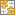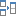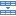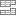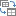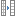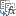# Excel COUNTBLANK and other functions to count empty cells

The tutorial discusses the syntax and basic uses of the COUNTBLANK function to count the number of blank cells in Excel.

In a couple of recent posts, we've discussed different ways to identify blank cells and highlight blanks in Excel. In some situations, however, you may want to know how many cells do not have anything in them. Microsoft Excel has a special function for this too. This tutorial will show you the fastest and most convenient methods to get the number of empty cells in a range as well as totally blank rows.

## Excel COUNTBLANK function

The COUNTBLANK function in Excel is designed to count empty cells in a specified range. It belongs to the category of Statistical functions and is available in all versions of Excel for Office 365, Excel 2019, Excel 2016, Excel 2013, Excel 2010, and Excel 2007.

The syntax of this function is very straightforward and requires just one argument:

COUNTBLANK(range)

Where range is the range of cells in which blanks are to be counted.

Here is an example of the COUNTBLANK formula in Excel in its simplest form:

`=COUNTBLANK(A2:D2)`

The formula, entered in E2 and copied down to E7, determines the number of empty cells in columns A through D in each row and returns these results: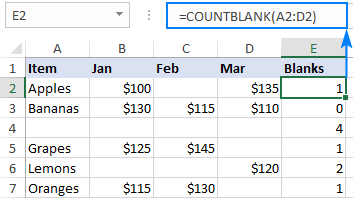Tip. To count non-blank cells in Excel, use the COUNTA function.

### COUNTBLANK function - 3 things to remember

To effectively use an Excel formula for counting blank cells, it is important to understand what cells the COUNTBLANK function considers as "blanks".

1. Cells that contain any text, numbers, dates, logical values or errors are not counted.
2. Cells containing zeros are considered non-blank and are not counted.
3. Cells containing formulas that return empty strings ("") are considered blank and are counted.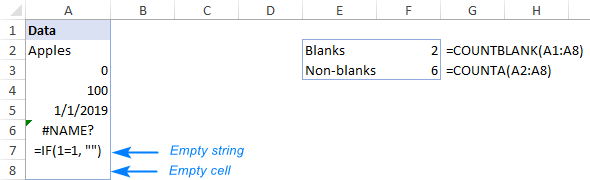Looking at the screenshot above, please notice that cell A7 containing a formula that returns an empty string is counted twice:

• COUNTBLANK considers a zero-length string as an empty cell because it appears blank.
• COUNTA treats a zero-length string as a non-empty cell because it actually contains a formula.

That may sound a bit illogical, but Excel does work this way :)

## How to count blank cells in Excel - formula examples

COUNTBLANK is the most convenient but not the only way to count empty cells in Excel. The following examples demonstrate a few other methods and explain which formula is best to be used in which scenario.

### Count blank cells in range with COUNTBLANK

Whenever you need to count blanks in Excel, COUNTBLANK is the first function to try.

For example, to get the number of empty cells in each row in the table below, we enter the following formula in F2:

`=COUNTBLANK(A2:E2)`

As we use relative references for the range, we can simply drag the formula down and the references will adjust automatically for each row, producing the following result: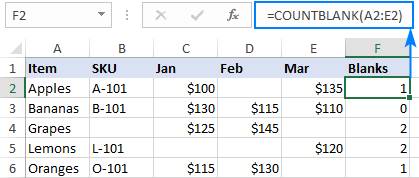### How to count blank cells in Excel using COUNTIFS or COUNTIF

Another way to count empty cells in Excel is to use the COUNTIF or COUNTIFS function or with an empty string ("") as the criteria.

In our case, the formulas would go as follows:

`=COUNTIF(B2:E2, "")`

Or

`=COUNTIFS(B2:E2, "")`

As you can see in the screenshot below, the results of COUNTIFS are exactly the same as those of COUNTBLANK, so which formula to use in this scenario is a matter of your personal preference.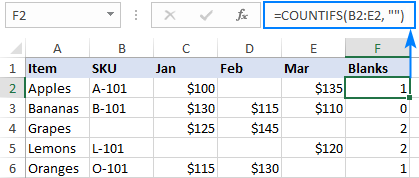### Count blank cells with condition

In a situation, when you want to count empty cells based on some condition, COUNTIFS is the right function to use as its syntax provides for multiple criteria.

For instance, to determine the number of cells that have "Apples" in column A and blanks in column C, use this formula:

`=COUNTIFS(A2:A9, "apples", C2:C9, "")`

Or input the condition in a predefined cell, say F1, and refer to that cell as the criteria:

`=COUNTIFS(A2:A9, F1, C2:C9, "")`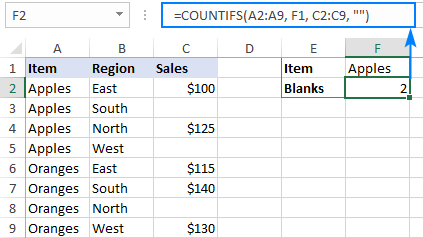### IF COUNTBLANK in Excel

In some cases, you may need not just count blank cells in a range, but take some action depending on whether there are any empty cells or not.

Although there is no built-in IF COUNTBLANK function in Excel, you can easily make your own formula by using the IF and COUNTBLANK functions together. Here's how:

• Check if the blanks count equals zero and put this expression in the logical test of IF:
`COUNTBLANK(B2:D2)=0`
• If the logical test evaluates to TRUE, output "No blanks".
• If the logical test evaluates to FALSE, output "Blanks".

The complete formula takes this shape:

`=IF(COUNTBLANK(B2:D2)=0, "No blanks", "Blanks")`

As the result, the formula identifies all the rows where one or more values are missing: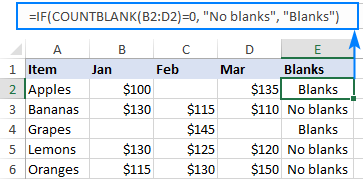Or you can run another function depending on the blanks count. For instance, if there are no empty cells in the range B2:D2 (i.e. if COUNTBLANK returns 0), then sum the values, otherwise return "Blanks":

`=IF(COUNTBLANK(B2:D2)=0, SUM(B2:D2), "Blanks")`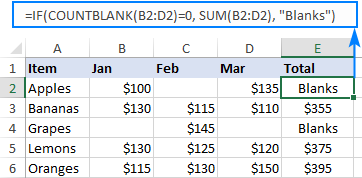### How to count blank rows in Excel

Supposing you have a table in which some rows contain information while other rows are totally blank. The question is - how do you get the number of rows that do not contain anything in them?

The easiest solution that comes to mind is to add a helper column and fill it with the Excel COUNTBLANK formula that finds the number of blank cells in each row:

`=COUNTBLANK(A2:E2)`

And then, use the COUNTIF function to find out in how many rows all the cells are blank. Since our source table contains 5 columns (A through E), we count the rows that have 5 empty cells:

`=COUNTIF(F2:F8, 5))`

Instead of "hardcoding" the number of columns, you can use the COLUMNS function to calculate it automatically:

`=COUNTIF(F2:F8, COLUMNS(A2:E2))`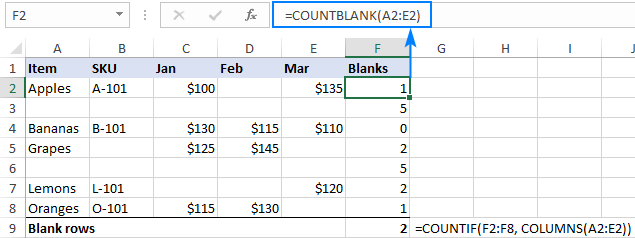If you do not want to mangle the structure of your beautifully designed worksheet, you can achieve the same result with a lot more complex formula that does not however require any helper columns nor even array entering:

`=SUM(--(MMULT(--(A2:E8<>""), ROW(INDIRECT("A1:A"&COLUMNS(A2:E8))))=0))`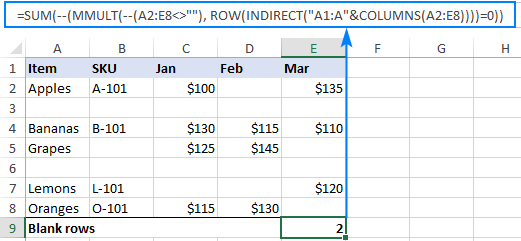Working from the inside out, here's what the formula does:

• First, you check the entire range for non-blank cells by using the expression like A2:E8<>"", and then coerce the returned logical values of TRUE and FALSE to 1's and 0's by using the double unary operator (--). The result of this operation is a two-dimensional array of ones (non-blanks) and zeros (blanks).
• The purpose of the ROW part is to generate a vertical array of numeric non-zero values, in which the number of elements equals to the number of columns of the range. In our case, the range consists of 5 columns (A2:E8), so we get this array: {1;2;3;4;5}
• The MMULT function calculates the matrix product of above arrays and produces a result like: {11;0;15;8;0;8;10}. In this array, the only thing that matters for us is 0 values that represent the rows where all cells are blank.
• Finally, you compare each element of the above array against zero, coerce TRUE and FALSE to 1 and 0, and then sum the elements of this final array: {0;1;0;0;1;0;0}. Keeping in mind that 1's correspond to blank rows, you get the desired result.

If the above formula seems too difficult for you to comprehend, you may like this one better:

`=SUM(--(COUNTIF(INDIRECT("A"&ROW(A2:A8) & ":E"&ROW(A2:A8)), "<>"&"")=0))`

Here, you use the COUNTIF function to find how many non-blank cells there are in each row, and INDIRECT "feeds" the rows to COUNTIF one by one. The result of this operation is an array like {4;0;5;3;0;3;4}. A check for 0, transforms the above array to {0;1;0;0;1;0;0} where 1's represent blank rows, so you just need to add them up.

### Count truly blank cells excluding empty strings

In all the previous examples, we were counting blank cells including those that only appear blank but, in reality, contain empty strings ("") returned by some formulas. In case you'd like to exclude zero-length strings from the result, you can use this generic formula:

ROWS(range) * COLUMNS(range) - COUNTA(range)

What the formula does is to multiply the number of rows by the number of columns to get the total of cells in the range, from which you subtract the number of non-blanks returned by COUNTA. As you may remember, the Excel COUNTA function considers empty strings as non-blank cells, so they won't be included in the final result.

For example, to determine how many absolutely empty cells there are in the range A2:A8, here's the formula to use:

`=ROWS(A2:A8) * COLUMNS(A2:A8) - COUNTA(A2:A8)`

The screenshot below shows the result: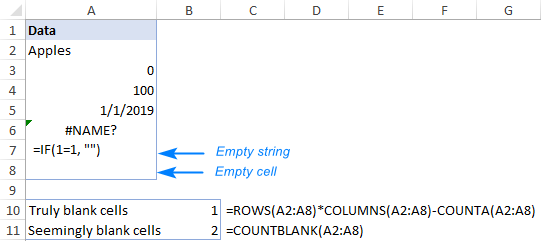That's how to count empty cells in Excel. I thank you for reading and hope to see you on our blog next week!

Count blank cells formula examples

### 2 responses to "Excel COUNTBLANK and other functions to count empty cells"

1.Richa Reddy says:

To count the number of blanks in each row for a particular column(E2) in all the sheets('17-Mar' till '12-Jun') in workbook in excel.

•Alexander Trifuntov (Ablebits.com Team) says:

Hello!
The COUNTBLANK function cannot count the number of empty cells using a 3D reference. Therefore, it must be used in the usual way, as described in this tutorial above.

Ultimate Suite for Microsoft Excel